# Next >>

## Comparing two cases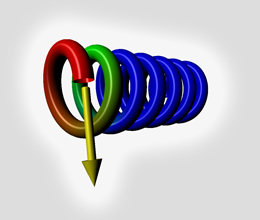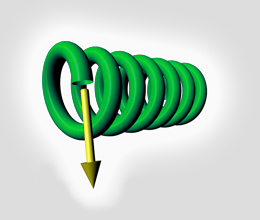A rope is applied around a pole with a lot of friction. The very first loop absorbs the tension. The pressure is localized and the rest of the rope is almost free of any mechanical constraint. No tension is propagated to the end of the rope. A rope is applied around a pole with zero friction (Of course this is just an assumption). The constraint is transmitted along the rope from one end to the other. The pressure is perfectly distributed.Quick links   << The perfect rope : the O-link

### A true but uncomplete assertion

It is often considered as safer to make numerous turns around the arm, the ankles or the wrists. BUT THIS IS NOT ENOUGH to lower the pressure applied by the rope. Indeed you must not neglect the friction in the evaluation of pressure. Reading these lines will help you to make your bondage safer. In this one-page study, we will try to quantify the transmission of the force along a rope surrounding a pole. You will see at the end of this development, that –in the case of significant friction– the transmitted strength is lost exponentially with number of turns while the pressure concentrates in the very first loops.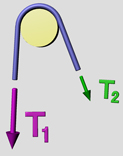### The equations How you can determin the effect of the loops.

To the first order, the normal strength N at a given contact point (i.e. angle coordinate q) between the pole and the rope is proportional to the tension in the rope. The factor a contains curvature information and other physical properties of the rope. (We may also add here some information on external forces applied to the rope towards the pole).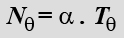Furthermore, the contribution to the decrease of the tension is the longitudinal friction force applied by the pole to the rope. It is proportional to the normal strength, to the surface in contact and to the friction coefficient f. Notice that dT is a reduction in force and thus is negative.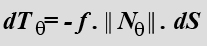We can express the elementary surface dS as the product of its width and of its length.
b is the flattening factor of the rope, and the width of the contact surface is related to the rope diameter by the product w = b.d. The length of the elementary contact surface is related to the radius of the pole r by the equation L = r.dq, where dq is the elementary change in angle around the pole. (we consider that d<<r and neglect the quantity d/2 in the total curvature d/2 + r)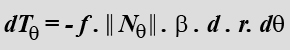Then we substitute N by its expression to obtain :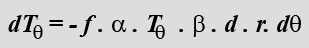Finally all constant factors are summarized in the single value A. Note that the higher the friction coefficient, the bigger the constant A would be.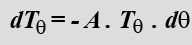We can now calculate the change in strength along the path q1 to q2.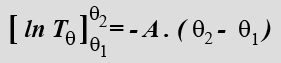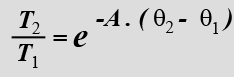G represents the number of turns around the pole. For most of values of A, the pressure is concentrated in the very first loops.

### Lessons learned

In conclusion, we have observed that the tension along the path of the rope drops exponentially. In other words, 95% of the strength is transmitted in the two first loops around the pole…Further rigging is strictly unnecessary. Numerous turns are not the guarantee of a lower pressure. That would only be true for a theoretical situation (no friction), which of course never happens.

### How does it apply to rope bondage?In order to achieve a uniform distribution of the strength you have first to make your tying perfect. i.e. you need to make your bondage rope look like the O link shown in introduction. It must be closed : Your knots must be perfectly tight. Then you have to lower the friction as much as possible: chose a rope that slips well on the skin. Of course, the knots you are trying to tight will not hold if you chose a very slippery material like silk... The trade off is up to you, but fore safety reasons, please mind the first loop and release some pressure frome time to time!
 Solutions Hopefully, there are some common sense solutions to distribute the pressure to all the turns: You will only need to slip your finger at the contact surface, between the skin and the rope. This will relax the most tightened loops and distribute the tension will be transmitted to the lose turns. I also tend to use silk ropes but again, it may cause your whole bondage to untie due to unsufficient friction of the rope onto itself.

### Other reasons for doubling the ropes

Maybe you have noticed already that at a given point, the number of rope ends is an even number? This is quite obvious: each rope arriving in a point goes also away from this point. As a result, a knot has an even number of ends whatever the number of ropes that are used...
Read more in the dedicated section >>

# Next >>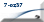About me | Site Map | Copyright Policy | Contact me | ©2008 7-oz37.com